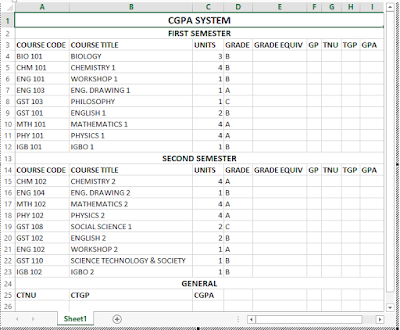## Friday, 4 November 2016

You can use Microsoft Excel to calculate your Cumulative Grade Point Average for a session or your Grade point Average (GPA) for a semester. Excel reduces the stress for you. All you need to do is to insert the insert the correct syntax in the correct column, then excel performs the calculations for you.

This is the Part 4 of chapter 2 of Excel tutorial. You are advised to read the first two parts:

In this part 4 of chapter to of Excel tutorial, I will explicitly explain how to calculate your academic CGPA with practical illustrations.

The illustration in this section is for a five-point grade system. But the procedure is the same for other grade systems. Also in the example below, I assumed you offered nine courses both in first and second semester. If the number of courses you offered is less or more than nine, don’t panic. All you need to do is to adjust the cell references in the syntax of the function used.

The two main functions needed to design a CGPA System are the IF and SUM functions.

## Definitions of Some CGPA Terms Used in this Illustration

### 1. UNITS:

This can also be referred to as Credit Unit. It is the credit load given to a course by the university management. Different courses have different credit units.

There are different types of grade system. The two most popular grade systems are the Five-Point Grade System and the Three-Point Grade System.
In the Five-Point Grade System, there are 6 grade letters, but only five out of the six letters are valid. The grade letters A, B, C, D, E, F represent the values 5, 4, 3, 2, 1, 0 respectively.

The letter F is not valid since zero which is its value is an invalid number.
In the Three-Point Grade System, there are 4 grade letters where only three out of the four grade letters are valid.

As the name implies, it is the numerical equivalence of the grade letter. For example, in a Five-Point Grade System, the equivalence of the letters A, B, C, D, E, F are 5, 4, 3, 2, 1, 0 .

### 4. GP:

This means Grade Point. It is the value of the Grade letter equivalence multiplied by the Credit Unit.

### 5. TNU:

This means Total Number of Units. It is the sum of the credit units of all the courses offered in a semester.

### 6. TGP:

This means Total Grade Point. It is the sum of the grade points you made in all the courses offered in a semester.

### 7. GPA:

This means Grade Point Average. It is the value of the TGP divided by the TNU.

### 8. CTNU:

This means Cumulative Total Number of Units. It is the sum of the TNU for first and second semester.

### 9. CTGP:

This means Cumulative Total Grade Point. It is the sum of the TGP for first and second semester.

### 10. CGPA:

This means Cumulative Grade Point Average. It is the value of the TGP divided by the TNU for the academic session.

## Designing the CGPA System in MS-Excel

It is now time to design the CGPA System.
Prepare the three tables - first semester, second semester and general tables on MS-Excel worksheet as shown in the figure below.## Syntaxes for the CGPA System

### For the First Semester Table

Type in the following syntaxes in the specified cells:

Position your cell pointer in cell E4 and type the syntax:
=IF(D4="A",5,IF(D4="B",4,IF(D4="C",3,IF(D4="D",2,IF(D4="E",1,IF(D4=F,0)))))) press Enter. Use the auto fill handle to generate the values for the subsequent cells in the column.

2. For the GP;
Position your cell pointer in cell F4 and type the syntax:
=C4*E4 press Enter. Use the auto fill handle to generate the values for the subsequent cells in the column.

3. For the TNU;
Position your cell pointer in cell G4 and type the syntax:
=SUM(C4:C12) press Enter.

4. For the TGP;
Position your cell pointer in cell H4 and type the syntax:
=SUM(F4:F12) press Enter.

5. For the GPA;
Position your cell pointer in cell I4 and type the syntax:
=H4/G4 press Enter.

### For the Second Semester Table

Type in the following syntaxes in the specified cells:

Position your cell pointer in cell E15 and type the syntax:
=IF(D15="A",5,IF(D15="B",4,IF(D15="C",3,IF(D15="D",2,IF(D15="E",1,IF(D15=F,0)))))) press Enter. Use the auto fill handle to generate the values for the subsequent cells in the column.

2. For the GP;
Position your cell pointer in cell F15 and type the syntax:
=C15*E15 press Enter. Use the auto fill handle to generate the values for the subsequent cells in the column.

3. For the TNU;
Position your cell pointer in cell G15 and type the syntax:
=SUM(C15:C23) press Enter.

4. For the TGP;
Position your cell pointer in cell H15 and type the syntax:
=SUM(F15:F23) press Enter.

5. For the GPA;
Position your cell pointer in cell I15 and type the syntax:
=H15/G15 press Enter.

### For the General Table

Type in the following syntaxes in the specified cells:
1. For the CTNU;
Position your cell pointer in cell A26 and type the syntax:
=G4+G15 press Enter.

2. For the CTGP;
Position your cell pointer in cell B26 and type the syntax:
=H4+H15 press Enter.

3. For the CGPA;
Position your cell pointer in cell C26 and type the syntax:
=B26/A26 press Enter.

Your completed CGPA System should resemble the one shown in the figure below.Congratulations! You have built a system for calculating your CGPA for an academic session using Microsoft Excel.

This is the end of the part 4 of chapter 2.
Now go over to Part 5:

Comment below if you are hooked up along the installation process.

1.I would make a column for each of the following:
Semester
Class
Credits

2.